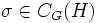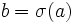# Finitary symmetric group is centralizer-free in symmetric group

(diff) ← Older revision | Latest revision (diff) | Newer revision → (diff)
This article gives the statement, and possibly proof, of a particular subgroup or type of subgroup (namely, Finitary symmetric group (?)) satisfying a particular subgroup property (namely, Centralizer-free subgroup (?)) in a particular group or type of group (namely, Symmetric group (?)).

## Statement

### For the symmetric group, plain

Let$A$ be a set with at least three elements. Let$\operatorname{Sym}(A)$ denote the symmetric group on$A$ (the group of all permutations on$A$). Then,$\operatorname{Sym}(A)$ is trivial.

### For the symmetric group and finitary symmetric group

Let$A$ be a set with at least three elements. Let$\operatorname{Sym}(A)$ denote the symmetric group on$A$ (the group of all permutations on$A$, and$\operatorname{FSym}(A)$ denote the subgroup comprising all finitary permutations -- permutations moving only finitely many elements. Then the centralizer of$\operatorname{FSym}(A)$ is$\operatorname{Sym}(A)$ is the trivial group. In other words, no non-identity permutation commutes with every finitary permutation.

### Relation between the two formulations

The second formulation is stronger than the first. For finite sets, the two formulations are precisely the same.

## Proof

Given: A set$A$ with at least three elements.$G = \operatorname{Sym}(A)$ is the group of all permutations of$A$, and$H = \operatorname{FSym}(A)$ is the subgroup comprising finitary permutations.

To prove:$C_G(H)$ is the trivial group.

Proof: Suppose$\sigma \in C_G(H)$ and$a \in A$. Our goal is to show that$\sigma(a) = a$.

Since$A$ has at least three elements, we can find a$b \in A$ such that$b \ne a$ and$b \ne \sigma(a)$. Let$\tau$ be the transposition$(a,b)$. Then, we have:$\sigma\tau\sigma^{-1} = \tau$.

On the other hand, we know that:$\sigma\tau\sigma^{-1} = (\sigma(a),\sigma(b))$.

Thus, the transposition$(a,b)$ and the transposition$(\sigma(a),\sigma(b))$ are equal. This forces$\{a,b\} = \{ \sigma(a),\sigma(b)\}$, so either$a = \sigma(a)$ or$b = \sigma(a)$. The latter case was ruled out by choice of$b$, so$a = \sigma(a)$, completing the proof.